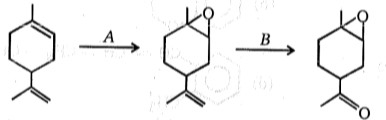Reagent (A) and (B) in the above reaction are:

(a) A = RCO3H, B = H2O2                         (b) A = RCO3H, B = HIO4

(c) A = RCO3H, B = O3                             (d) A = O3, B = RCO3H

High Yielding Test Series + Question Bank - NEET 2020

Difficulty Level:

Rank the following in the increasing order of rate of reaction with HBr.

(P)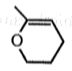(Q)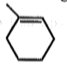(R)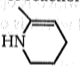(a) R>P>Q

(b) R>Q>P

(c) P>R>S

(d) P>S>R

High Yielding Test Series + Question Bank - NEET 2020

Difficulty Level:

Which of the following reactions generates the major product ? Ignore stereoisomerism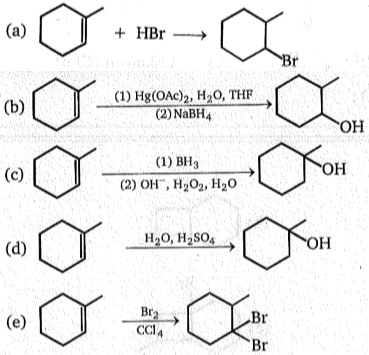High Yielding Test Series + Question Bank - NEET 2020

Difficulty Level:

In the given selective hydrogenation which combination is incorrect ?

1.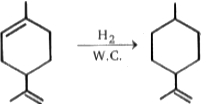(w.c. = wilkinsons catalyst)

2.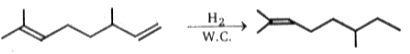(w.c. = wilkinsons catalyst)

3.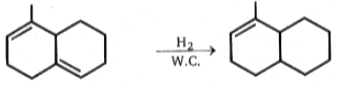4.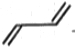$\underset{∆}{\overset{{\mathrm{H}}_{2}}{\to }}C{H}_{2}-CH=CH-C{H}_{3}$

High Yielding Test Series + Question Bank - NEET 2020

Difficulty Level: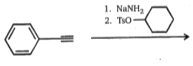$\left(\mathrm{A}\right)\stackrel{Na/N{H}_{3}\left(l\right)}{\to }\left(B\right)\stackrel{MCBPA}{\to }\left(C\right)$

Compound (C) in above sequence of reaction is:

1.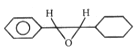2.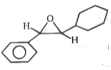3.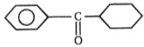4.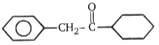High Yielding Test Series + Question Bank - NEET 2020

Difficulty Level: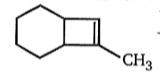$\underset{{\mathrm{R}}_{2}{\mathrm{O}}_{2}/\mathrm{hv}}{\overset{\mathrm{HBr}}{\to }}$(A);

Major product (A) is:

(a)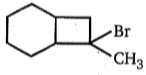(b)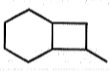(c)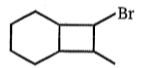(d)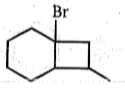Concept Questions :-

Alkanes, Alkenes and Alkynes - Chemical Properties
High Yielding Test Series + Question Bank - NEET 2020

Difficulty Level:

In the reaction given below, the product would be :-
OH
H3O+                l
CH3-CH=CH-CH3→CH3-CH2-CH-CH3

(a)a mixture of diastereomers
(b)optically active
(c)optically pure enantiomer
(d)a recemic mixture

High Yielding Test Series + Question Bank - NEET 2020

Difficulty Level: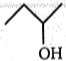$\underset{∆}{\overset{{\mathrm{H}}^{+}}{\to }}$Possible products $\stackrel{{\mathrm{Br}}_{2}/{\mathrm{CCl}}_{4}}{\to }$(y) products

The number of possible products for x and y is:

(a) 2,4

(b) 3,5

(c) 3,6

(d) 3,4

High Yielding Test Series + Question Bank - NEET 2020

Difficulty Level: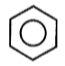Product (C) of the above reaction is :

Concept Questions :-

Aromatic Hydrocarbons - Benzene - Structure, Preparation & Chemical Reactions
High Yielding Test Series + Question Bank - NEET 2020

Difficulty Level: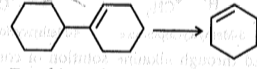To achieve above conversion, the reagents used will be:

(a) O3/H2O2, HO-/$∆$                                 (b) HBr, alc. KOH, O3, LiAlH4, H+/$∆$

(c)  HBr, t-BuOK, O3, KMnO4$∆$